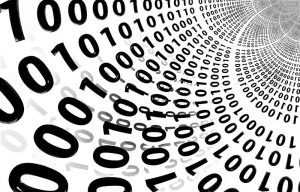Mathematics

Binary system

The binary system, or base system 2, is a numerical system that uses only two digits: 0 and 1. Computers operate by means of a binary system, which means that they can store data and perform calculations using only zeros and ones. The term is also used to refer to any digital coding and decoding system in which there are two exact possible states. In digital data memory, storage, processing and communications, the values 0, which is referred to as low; and 1 which may also be referred to as "high". A bit, which is the name given to the abbreviation of a binary digit, is the smallest unit of data that can be found on a computer; each bit has a unique value of 1 or 0. Executable programs are often identified as binary files and receive a ".bin" file name extension. Programmers often call them binary executable files.Related topics

What is the binary system?

The binary system is a set of components or elements that interact and relate to each other and that serves to represent texts, data or to process different instructions in a computer or a computer device.

What is the binary system for?

Nowadays, the binary system is one of the most significant, because its popularity lies in the use given to it in computers. As these equipment internally, work with two different degrees of voltage, the binary system is used to indicate the shutdown, the low power, “zero volts” or the inhibited stage. The binary numbering system has different uses today ranging from microprocessor programming, data transfer, information encryption to digital communication, electronics and other computer-related areas. It is also important in the field of electronics and current technology, as they are present in most of the artifacts we use daily.

Characteristics

The main features of the binary system are as follows:

• It uses only two digits, zero and one.
• Each digit has a different value depending on its position.
• The value of each position is the same as that of a base power 2.
• It is used in all types of computers because they work with internal voltage drops.

History

The mathematician of Indian origin, Pingala, presented for the first time a description of a binary numbering system in the third century B.C., in which he represented the numbers from 1 to 8 with a sequence, consisting of 8 trigrams and 64 hexagrams, analogous to binary numbers with an accuracy of 3 and 6 bits. The binary system was modernized by Gottfried Leibniz, in the 17th century, through his written work known as Explication de l’Arithmétique Binaire. This modernized Leibniz system used the 0 and 1, as it is maintained today. In 1854, the British mathematician George Boole detailed a logical system that would later be called Boolean algebra which was essential for the development of the binary system, mainly in its application to electronic circuits.

How the binary system works

The binary system is based on the representation of quantities using the numbers 1 and 0. Therefore, its base is 2, which is the number of digits that a system has. Each digit in this system is called a bit. These numbers start with 0 and then 1 followed by 1 and now we would have to move on to the next number, which would already be two digits because there are no more one-digit binary numbers. The next binary number, therefore, would be to combine 1 with 0, i.e. 10, the next would be the number 11. Made all possible combinations of 2-digit binary numbers, we have no more combinations, so we build the 3-digit ones, and so on.

Application

The uses and applications that can be given to the binary system are:

• Computing
• Electronics
• Electricity

Conversion from binary system to decimal

In order to convert a binary number into a decimal, we need to multiply by the number two numbers from the first binary term and to the result of that multiplication we must add 1, later, that result we must multiply by two and add the next binary and so on until we have a digit which only must be added and not multiplied.

Examples

• 0: 0
• 1: 1
• 10: 2
• 11: 3
• 100: 4
• 101: 5
• 110: 6
• 111: 7
Written by Gabriela Briceño V.(2 votos, promedio: 5.00 de 5)Loading...